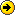Engineer's Allure
This Forum is the only Pakistan's platform dedicated to engineering & science disciplines. So join us & open up channels for technical discussions. If you are a Professional Engineer or a Teacher Contact Us ...& you may be accepted as Forum ModeratorGet the most out of our technical forum using these tutorials:

General Forum Usage
Insert real-time Math Equations
Insert Streaming Documents
Latest topics
» Tough Life of a Resin Bead
by ioncube Fri Jul 19, 2013 8:16 pm

» Final Year Projects Suggestions
by ioncube Fri Jul 19, 2013 2:48 pm

» Tracking Natural Gas Via Flowmeters
by ioncube Sat Sep 08, 2012 11:04 pm

» Maximising Heat-Transfer Fluid Life
by ioncube Thu Aug 23, 2012 6:16 pm

 So now we will learn the technique that is mostly missing from many scientific platforms: The ability to generate flexible & real-life Math equations. Not the conventional image files that you can't edit afterwards; but a live equation that can be customised as many times as user wishes. Application neededMathType Available for both Windows & MacOS systems. Runs also in Linux under Wine How to get it : You can do a search at Mediafire or you can search at FilesTube No Luck : Manually Contact us. Using MathType The interface is as easy as its get, click on the symbols, fill the blanks & your equation is ready in seconds.Customising Application. Pay attention. After you open Mathtype configure these settingsAll is set, preferences will be saved permanently. Import MathType Equations to this forum. Try to insert some calculus equation or a matrix or something...maths.I just selected some very complex math symbols.Select the whole code via highlighting or using Ctrl+A. Then copy it using Ctrl+C. Then paste it in our forum message editor & hit preview.Congratz...You did it. To save Bandwidth you willn't see equations in 'preview' mode; but they WILL appear when you 'send' the post...can be edited latter if required Understanding Latex code You didn't copied symbols!! : When you selected the whole code in MathType & copied it, basically all the symbols get 'translated' in special Latex language or code. This code if you can observe is enclosed by 'two' $signs. And after the first$ sign follows a backslask \. These are the special syntax used to identify latex code in our forum. This code then re-translates to the MathType image using javascript parsing. Some code errors : We have discovered that when we use some trignometric functions, MathType output translation isn't right. After the first $tag, instead of \ there occurs { which has to be manually eliminated Utilising other Latex codes Get a Latex Editor for offline usage: Say for instance you posted a quiestion answer to which is given some following equations: Now if you hover your mouse cursor over the quation you can see the Latex code; but how do you select it? If you select whole of the equation (via dragging mouse cursor) & select copy (& not copy image); then paste it any latex enable editor e.g Mathematica, MathType....you will get the required equation. Plus you can also use Microsoft Office Word (with MathType plugin installed) to compose all the equations in your research paper or assisgnment.Try selecting » copying » pasting following examples in Latex enabled ediotrs...starting with the MathType.MathType supports all popular offices & CAD designing applications. To know if it supports your application click here.The above method of ‘selecting by highlighting’ isn’t working in Opera browser. Though you can save equation as an image. Thus we recommend that beside publishing regular equation enclose by$ tags, user also post the original code (without $tags) in the message body. Like this: Or you can try another approach to this problem:$$J_\alpha(x) = \sum_{m=0}^\infty \frac{(-1)^m}{m! \, \Gamma(m + \alpha + 1)}{\left({\frac{x}{2}}\right)}^{2 m + \alpha}$$ $$\iiint f(x,y,z)\,dx\,dy\,dz$$ $$A \alpha B \beta \Gamma \gamma \Delta \delta \dots \Phi \phi X \chi \Psi \psi \Omega \omega$$ $$C H_4$$$V = \left( \begin{array}{ccc} 1-\frac{1}{2}\lambda^2 & \lambda & A\lambda^3(\rho-i\eta) \\ -\lambda & 1-\frac{1}{2}\lambda^2 & A\lambda^2 \\ A\lambda^3(1-\rho-i\eta) & -A\lambda^2 & 1 \end{array} \right) + {\cal O}(\lambda^4)\$ $$\int_{0}^{1} x dx = \left[ \frac{1}{2}x^2 \right]_{0}^{1} = \frac{1}{2}$$